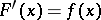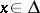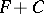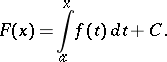# Indefinite integral

(diff) ← Older revision | Latest revision (diff) | Newer revision → (diff)

An integral(*)

of a given function of a single variable defined on some interval. It is the collection of all primitives of the given function on this interval. Ifis defined on an intervalof the real axis andis any primitive of it on, that is,for all, then any other primitive ofonis of the form, whereis a constant. Consequently, the indefinite integral (*) consists of all functions of the form.

The indefinite Lebesgue integral of a summable function onis the collection of all functions of the formIn this case the equalityholds, generally speaking, only almost-everywhere on.

An indefinite Lebesgue integral (in the wide sense) of a summable functiondefined on a measure spacewith measureis the name for the set functiondefined on the collection of all measurable setsin.

How to Cite This Entry:
Indefinite integral. Encyclopedia of Mathematics. URL: http://encyclopediaofmath.org/index.php?title=Indefinite_integral&oldid=11527
This article was adapted from an original article by L.D. Kudryavtsev (originator), which appeared in Encyclopedia of Mathematics - ISBN 1402006098. See original article